How PROC MCMC Works

By default, PROC MCMC uses the random walk Metropolis algorithm to obtain posterior samples. For details about the Metropolis algorithm, see the section Metropolis and Metropolis-Hastings Algorithms. For the actual implementation details of the Metropolis algorithm in PROC MCMC, such as the blocking of the parameters and tuning of the covariance matrices, see the section Tuning the Proposal Distribution. In some situations, PROC MCMC uses a conjugate updater (see the section Conjugate Sampling).

By default, PROC MCMC assumes that all observations in the data set are independent, and the logarithm of the posterior density is calculated as follows: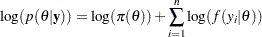where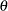is a parameter or a vector of parameters. The term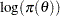is the sum of the log of the prior densities specified in the PRIOR and HYPERPRIOR statements. The term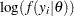is the log likelihood specified in the MODEL statement. The MODEL statement specifies the log likelihood for a single observation in the data set.

The statements in PROC MCMC are in many ways like DATA step statements; PROC MCMC evaluates every statement in order for each observation. The procedure cumulatively adds the log likelihood for each observation. Statements between the BEGINNODATA and ENDNODATA statements are evaluated only at the first and the last observations. At the last observation, the log of the prior and hyperprior distributions is added to the sum of the log likelihood to obtain the log of the posterior distribution.

With multiple PARMS statements (multiple blocks of parameters), PROC MCMC updates each block of parameters while holding the others constants. The procedure still steps through all of the programming statements to calculate the log of the posterior distribution, given the current or the proposed values of the updating block of parameters. In other words, the procedure does not calculate the conditional distribution explicitly for each block of parameters, and it uses the full joint distribution in the Metropolis step for every block update. If you want to model dependent data—that is,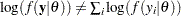—you can use the PROC option JOINTMODEL. See the section Modeling Joint Likelihood for more details.